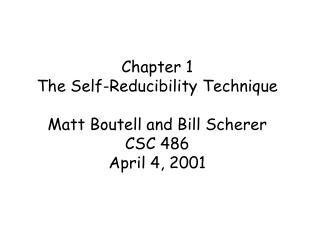# Chapter 1 The Self-Reducibility Technique Matt Boutell and Bill Scherer CSC 486 April 4, 2001 - PowerPoint PPT PresentationDownload PresentationChapter 1 The Self-Reducibility Technique Matt Boutell and Bill Scherer CSC 486 April 4, 2001

Chapter 1 The Self-Reducibility Technique Matt Boutell and Bill Scherer CSC 486 April 4, 2001
Download Presentation## Chapter 1 The Self-Reducibility Technique Matt Boutell and Bill Scherer CSC 486 April 4, 2001

- - - - - - - - - - - - - - - - - - - - - - - - - - - E N D - - - - - - - - - - - - - - - - - - - - - - - - - - -
##### Presentation Transcript

1. Chapter 1The Self-Reducibility TechniqueMatt Boutell and Bill SchererCSC 486April 4, 2001

2. Historical Perspective [Berman 1978]: P=NP   a tally set that is m-hard for NP [Mahaney 1982]: P=NP   a sparse set that is m-complete for NP [Ogiwara, Watanabe 1991]: today’s lecture p p

3. Proof Overview p Theorem: If  an NP btt-hard sparse set S, then P = NP. Technique: let L be an arbitrary language in NP. Then, using S and the reduction, we give a deterministic polynomial algorithm to decide L.

4. x Accept An Alternate Characterization of the Class NP A language LNP  [AP, polynomial p | x*, xL IFF (w)[wp(|x|)  x,wA]. x = input w = witness = certificate = accepting path: A = checking algorithm

5. x Accept Left Sets The left set, denoted Left[A,p], is {x,y | x*  yp(|x|)  (wp(|x|))[w lex y  x,wA]}. Note that having the left set non-empty  the existence of an accepting path.

6. Maximum Witnesses The Maximum Witness for some input x, denoted wmax(x), is max{y | yp(|x|)  x,yA]}. Deciding xL  Determining if wmax(x) is defined. (x*)(yp(|x|))[x,yLeft[A,p]  ylex wmax(x)]. (1.4)

7. Left[A,p]  NP Left[A,p]  NP (by guessing wmax(x)), so since S is NP-hard, Left[A,p] btt S via some function f. p

8. What does btt mean? p p Bounded truth table reductions, btt, are a type of reduction that uses a very weak form of oracle. Ak-ttB via some function f means that A = L(MB), where M is a deterministic polynomial machine that on input x precomputes up to k queries, asks them all in parallel, and uses a k-ary Boolean function to compute the output. v1v2 v3 … vk 0 0 0 … 0 Yes M: 0 0 0 … 1 No 1 1 1 … 1 No p

9. Back to the Proof… Let u be a pair x,y. Then with our reduction, uLeft[A,p]  S satisfies f(u). Now, with query strings v1, v2, v3, … vk, let (S(v1), S(v2), S(v3), … S(vk))  {Yes, No}; this line is a row in the truth table in our reduction to S. So f(u) is of the form , v1, v2, v3, … vk.

10. Intervals The trick we will use is to generate a polynomially bounded list of candidates for wmax(x). Once this list is generated, we can use brute force computation to see if any of these candidates are in fact witnesses. We do this by keeping track of a set of pair-wise disjoint intervals  in the range 0p(|X|)..1p(|X|), starting initially with the entire range.

11. wmax(x) The Interval Invariant xL  wmax(x)UII (1.5)

12. wmax(x) Last Definition: Covering Let 1, 2 be two collections of pair-wise disjoint intervals over p(|x|). Then 1 covers 2 with respect to x if: 1) (I2) (J1)[IJ] 2) wmax(x)  UI1 wmax(x)  UI2 0 1 2

13. wmax(x) Facts With Covering Let 1, 2, 3, 4 be sets of pair-wise disjoint intervals over p(|x|). Then (all with respect to x): 1) If the interval invariant holds for 1 and 2 is a cover of 1, it also holds for 2. 2) If 2 covers 1 and 3 covers 2, then 3 covers 1. 3) If 2 covers 1 and 4 covers 3, then 2U4 covers 1U3. 0 1 2

14. The Theorem, Restated p If  an NP btt-hard sparse set S, then P = NP.